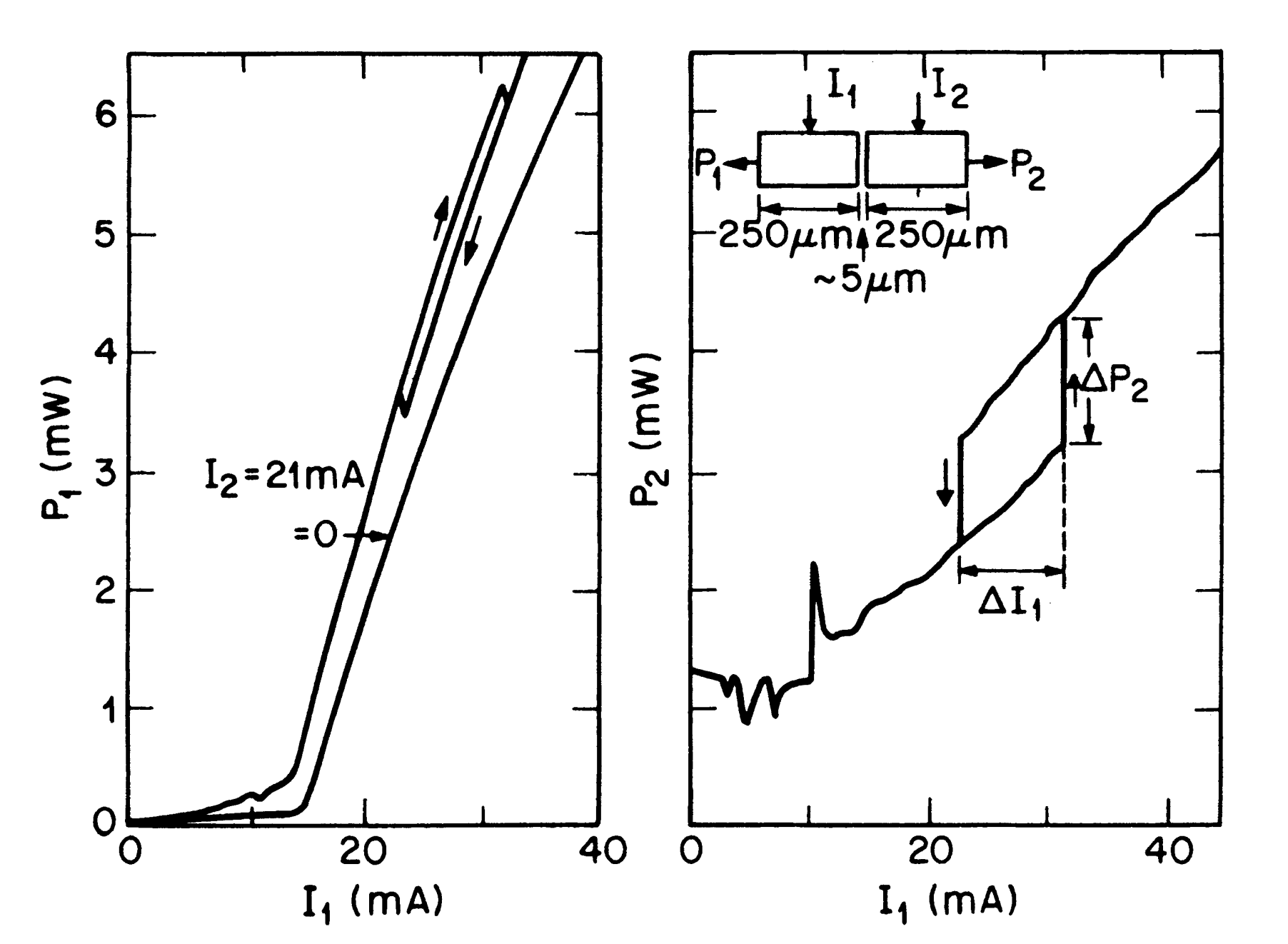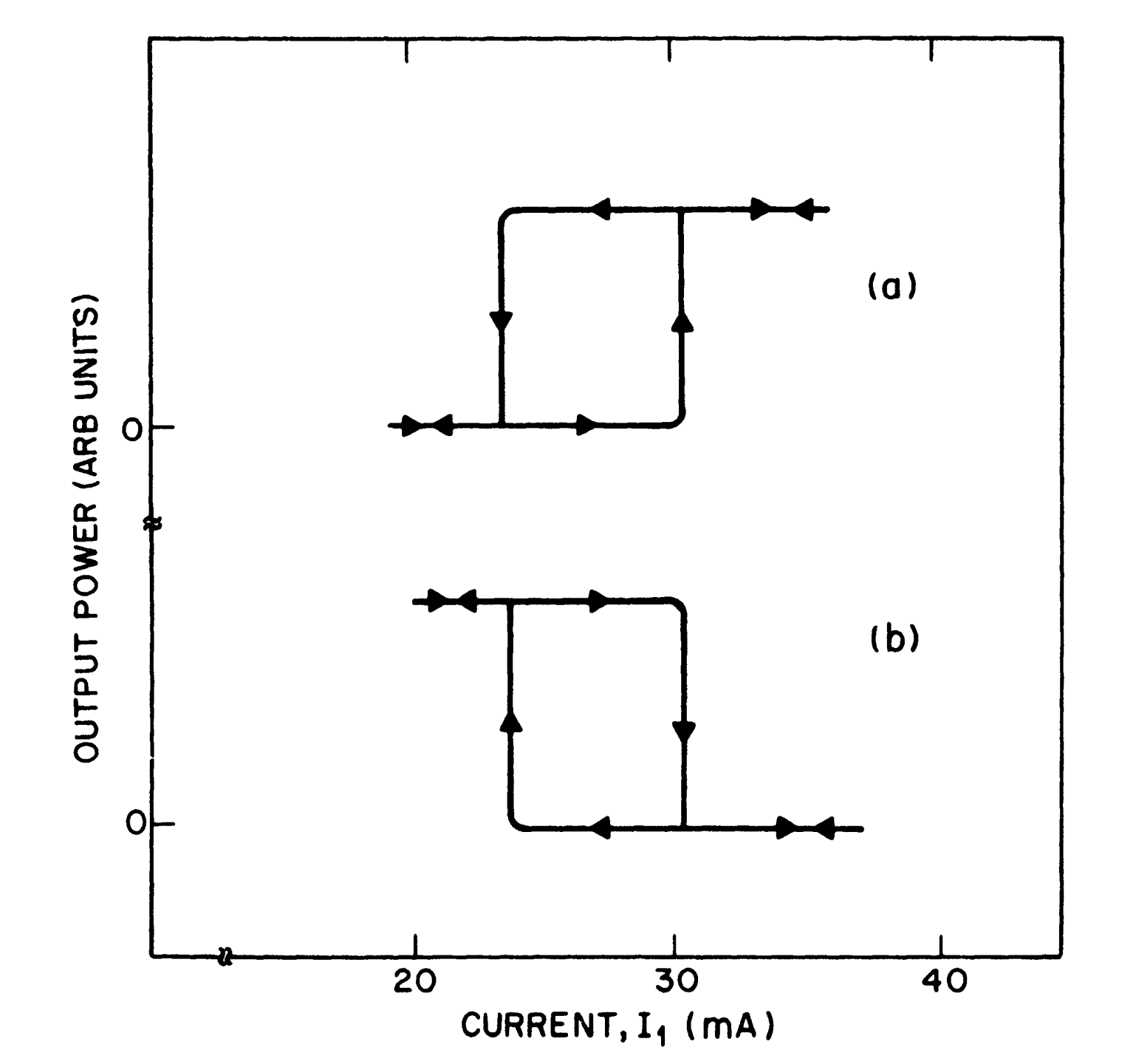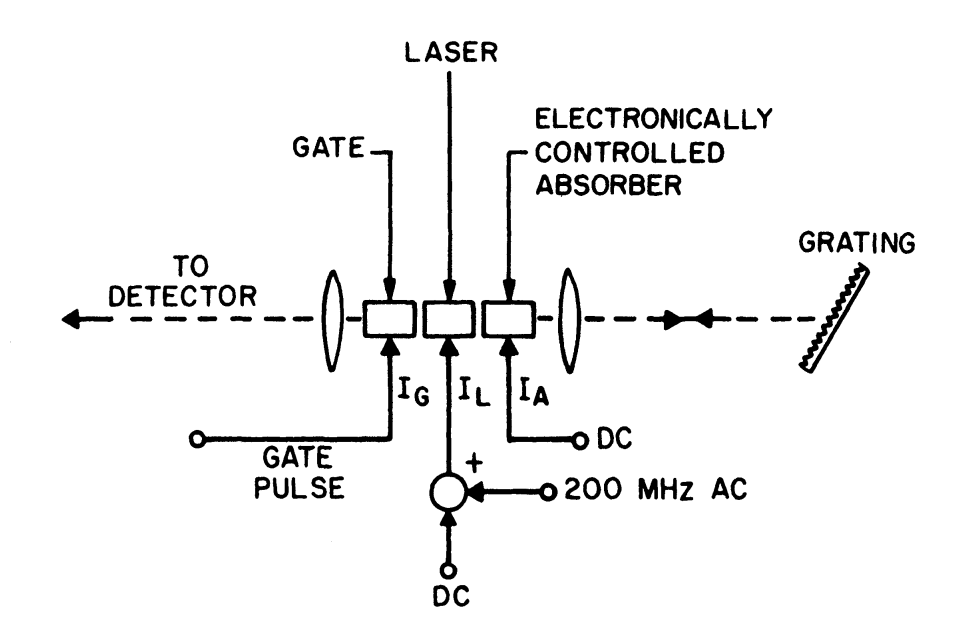# Diverse Applications of Coupled-Cavity Semiconductor Lasers

This is a continuation from the previous tutorial - operating characteristics of coupled-cavity semiconductor lasers.

In the operating characteristics of coupled-cavity semiconductor lasers tutorial the performance of coupled-cavity semiconductor lasers was discussed from the standpoint of their applications in optical fiber communications as a single-frequency optical source.

However, such lasers exhibit features that are useful for many other applications as well. This is particular true for active-active three-terminal devices, and many interesting applications of these have already been proposed an demonstrated.

In this tutorial we briefly describe some of the diverse applications of $$\text{C}^3$$ lasers.

## Optical Bistability

The phenomenon of optical bistability has drawn considerable attention for many years. An optical bistable system has two stable stationary states for some range of operating conditions and displays hysteresis when switched from one state to another by changing an externally controlled operating parameter.

Optical bistability in semiconductor lasers was proposed in 1964 and demonstrated soon thereafter. By applying different currents to different parts of the laser cavity, the laser was effectively (but not physically) separated into two sections. One section acted as a saturable absorber and led to bistable operation.

The method of inhomogeneous current injection and the associated bistability and self-pulsing phenomena have attracted considerable attention. In the saturable absorption mechanism, bistability occurs close to the lasing threshold over a limited current and temperature range.

$$\text{C}^3$$ lasers have been found to show a different kind of optical bistability, one not based on the phenomenon of saturable absorption.

In this scheme, bistability occurs when the two cavities are biased close to or above threshold. Furthermore, depending on the relative bias levels, two kinds of bistable behavior are observed.

In one case the total output power in a single longitudinal mode displays bistability and hysteresis.

In the other case the bistability is associated with a mode hop occurring in the $$\text{C}^3$$ laser with a change in the controller current. The powers associated with each longitudinal mode exhibit bistability, while the total power in both modes remains relatively constant.

In Figure 8-14 we show the bistable behavior for the case in which the $$\text{C}^3$$ laser maintains the same longitudinal mode.

When cavity 2 is unbiased, one observes usual $$L-I$$ characteristics. However, when $$I_2=21$$ mA and cavity 2 is biased above threshold ($$I_2^\text{th}=18$$ mA), hysteresis is observed in the output of both cavities. The largest hysteresis loop, however, occurs for $$P_2$$.

The kink-like behavior in $$P_2$$ when $$I_1\lt{I}_1^\text{th}$$ is due to mode hopping and is similar to that shown in Figure 8-8 [refer to the operating characteristics of coupled-cavity semiconductor lasers tutorial].

The hysteresis width $$\Delta{I}_1$$ depends on $$I_2$$ and is ~ 12 mA for $$I_2=21$$ mA. However, it increases to ~ 35 mA when $$I_2=24.5$$ mA. Such large hysteresis widths are useful in many applications.Figure 8-14.  Optical bistability in a $$\text{C}^3$$ laser for the configuration shown schematically in the inset. Output powers $$P_1$$ and $$P_2$$ from two facets are shown as functions of $$I_1$$ while $$I_2$$ is kept fixed.

A theoretical analysis of the bistable behavior seen in Figure 8-14 can be carried out using the single-mode rate equations (8-3-19) to (8-3-21) [refer to the coupled-cavity semiconductor lasers tutorial] generalized to account for the intercavity coupling.

Bistability is found to occur most readily when the coupling phase $$\theta=\pi/2$$. The hysteresis width depends on the coupling constant $$C$$, defined by Equation (8-3-6) [refer to the coupled-cavity semiconductor lasers tutorial], and on the relative biasing levels $$\bar{I}_n$$ [$$\bar{I}_n=I_n/I_n^\text{th}\;(n=1,2)$$] of the two cavities.

The physical origin of optical bistability can be understood by noting that, depending on the relative biasing levels, one cavity is optically pumped at the expense of the other.

When $$\bar{I}_1\lt\bar{I}_2$$, cavity 1 is optically pumped so that $$P_1\gt\bar{P}_1$$ and $$P_2\gt\bar{P}_2$$, where $$\bar{P}_1$$ and $$\bar{P}_2$$ are the powers expected in the absence of coupling.

When $$\bar{I}_1\gt\bar{I}_2$$, the opposite happens.

When $$\bar{I}_1\approx\bar{I}_2$$, both lasers compete since both are equally above threshold.

Depending on the direction in which $$\bar{I}_1$$ is increased, $$P_2$$ is below or above $$\bar{P}_2$$. This leads to the bistability and hysteresis seen in Figure 8-14.

We now consider spectral bistability occurring when the $$\text{C}^3$$ laser is made to switch from one longitudinal mode to the adjacent one by changing current $$I_1$$ in the controller section. Figure 8-15 shows the spectrally resolved output power at wavelengths corresponding to the two longitudinal modes.Figure 8-15.  Spectral bistability in a $$\text{C}^3$$ laser. Output power $$P_2$$ when (a) $$\lambda=1.5122$$  μm and (b) $$\lambda=1.5102$$ μm is shown as a function of $$I_1$$. See the inset of Figure 8-14 for notation.

In the bistable region the $$\text{C}^3$$ laser lases in either of two longitudinal modes but never in both simultaneously. Which of the two modes will lase depends on how the operating point is approached. In contrast to the result shown in Figure 8-14, the total output power in both modes is approximately constant while crossing the bistable region.

The physical origin of spectral bistability is different from that for the power bistability considered earlier.

When the currents in the two sections of a $$\text{C}^3$$ laser are varied, there are zones of single-mode operation in the $$I_1-I_2$$ plane. Depending on the coupling phase, these zones may or may not overlap.

It was found that when the coupling phase $$\theta$$ is negative, the zones overlap resulting in bistability. By contrast, for positive values of $$\theta$$ less than $$\pi/2$$, there are voids between the current zones, corresponding to unstable regions with no steady-state operation.

Optical bistability of $$\text{C}^3$$ lasers may be useful for a wide range of signal-processing functions such as optical limiting, optical switching, and optical logic operations.

Spectral bistability can also be useful in optical communication systems employing frequency-shift keying. If the laser is biased to operate in the middle of the hysteresis loop (see Figure 8-15) one or the other wavelength can be selected by applying a small modulation current to one cavity. A basic advantage of such a scheme is the fast switching from one state to another. Since the $$\text{C}^3$$ laser operates above threshold for both the on and off states, a switching time of less than 1 ns is easily obtained.

## Optoelectronic Logic

The ability of a $$\text{C}^3$$ laser to switch from one longitudinal mode to another when a short current pulse is applied to the controller section can be exploited for use in basic logic operations.

In a feasibility demonstration, the two longitudinal modes were selected by applying different current pulses to the two cavity sections. A grating was used to spectrally resolve the output. The dc bias was selected to operate the laser below but close to the threshold.

When a current pulse was applied to either of the cavities, the laser operated above threshold at one wavelength, say $$\lambda_1$$. However, when the two pulses were on simultaneously, the laser wavelength changed to a second wavelength, $$\lambda_2$$. By partially overlapping the two pulses, it was shown that the output at $$\lambda_1$$ and $$\lambda_2$$ exhibited characteristics of the basic logic operations.

The present device accepts electrical input to produce optical output and should be distinguished from optical bistable devices, where both the input and output are in the optical form.

## Optical Routing

A related application is to use a $$\text{C}^3$$ laser as a switching element for routing information among different channels. Again, this applicating takes advantage of the electronic tuning of $$\text{C}^3$$ lasers.

The route or channel is determined by the wavelength of the optical signal. The number of channels and the corresponding access times are determined by the number of discrete wavelengths over which the $$\text{C}^3$$ laser can be tuned and how fast the wavelength can be switched.

As we saw in the longitudinal mode control section of the operating characteristics of coupled-cavity semiconductor lasers tutorial, the number of longitudinal modes over which the $$\text{C}^3$$ laser can be tuned depends on the relative cavity lengths; up to 15 discrete modes can be used with a proper design.

The switching time depends on the biasing of the wavelength control section and is determined by the carrier lifetime when the controller is biased below threshold. In a feasibility demonstration, switching among four channels was achieved with a switching time of ~ 2 ns.

## Mode Locking

Mode locking is often used to obtain short optical pulses from a semiconductor laser. The output generally takes the form of a continuous train of short-pulses. For some applications it is desirable to be able to isolate a single pulse or a predetermined number of pulses by a suitable gating mechanism.

The use of a $$\text{C}^3$$ laser for this purpose has been demonstrated. The basic idea is to use one cavity as a gating diode that blocks the output of the mode-locked semiconductor laser until a current pulse is applied to decrease the absorption. A third cavity section acting as an electrically controlled absorber can also be incorporated.

Figure 8-16 shows schematically the experimental setup for the mode-locking scheme employing such a three-section semiconductor laser.Figure 8-16.  Schematic illustration of a mode-locked $$\text{C}^3$$ laser with three coupled sections for electronic gating and optical absorption control.

The middle section acts as the semiconductor laser, which is actively mode-locked with a 200-MHz sinusoidal current superimposed on the dc bias.

The cavity section between the semiconductor laser and the grating acts as an absorber whose absorption can be controlled by changing the current $$I_\text{A}$$.

The third section, on the other side of the semiconductor laser, is the gating element. When no current is applied to the gate ($$I_\text{G}=0$$), mode-locked pulses suffer large optical absorption and are absorbed before reaching the detector. For an appropriate value of $$I_\text{G}$$, the gate becomes optical transparent. By adjusting the duration of the gating pulse, the desired number of mode-locked pulses can be obtained. The width of each pulse can be adjusted by changing the absorber current $$I_\text{A}$$.

It is clear that the mode selectivity of a $$\text{C}^3$$ laser is not being used in this mode of operation.

Since the three sections are formed by cleaving a single laser chip, one obtains self-aligned absorbing sections whose absorption can be electrically controlled.

## Integrated Modulator

In an interesting application of three-section coupled-cavity devices, the third section is used as an external modulator that also acts as an amplifier.

Figure 8-17 shows the integrated device schematically.Figure 8-17.  Schematic illustration of a $$\text{C}^3$$ laser with an integrated external modulator that also acts as an amplifier.

The two shorter sections constitute a $$\text{C}^3$$ laser that operates continuously in a single longitudinal mode. The longer section acts as the amplifier-modulator. Its output facet is antireflection-coated to prevent the amplified signal from being reflected back into the laser cavity.

With no current applied to the amplifier section ($$I_1=0$$), the light emitted by the $$\text{C}^3$$ laser is absorbed inside this section. However, If $$I_1$$ is chosen to be sufficiently large, the light can be amplified. By modulating the amplifier current between zero and this value, pulsed output can be obtained while maintaining the single-frequency operation.

Experimentally an amplifier gain of 20 dB with an output power of 1 mW has been demonstrated. When used as an external modulator, optical pulses at 1-GHz repetition rate were obtained with a high on-off extinction ratio and an MSR of more than 20 dB.

In a related experiment one of the cavity sections of the $$\text{C}^3$$ laser was used as an intracavity modulator while the other section was modified to obtain Q switching.

This was accomplished by pumping only a part of the laser cavity; i.e., its electrode was subdivided into two parts. The unpumped region acts as a saturable absorber and can be used to Q switch the $$\text{C}^3$$ laser.

The intracavity modulator is useful to modulate these Q-switch light pulses after synchronizing the bit rate with the pulse repetition rate. The initial experiment has shown the feasibility of this scheme.

In these diverse applications of $$\text{C}^3$$ lasers, one cavity section is used as a semiconductor laser while the other acts as an absorber whose absorption can be electrically controlled. The chief advantage is that the absorber (or amplifier) is integrated and aligned with the semiconductor laser. With a proper biasing scheme this section can also be used as a detector.

The next tutorial discusses about quantum-well semiconductor lasers in detail.